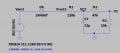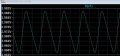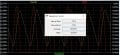# Design with half wave rectifier with filter capacitor

#### amineING

Joined Apr 8, 2020
7
I would like to have your opinion on some calculations I am doing on the unregulated output of a half-wave rectifier with filter capacitor.

The diagram is as follows:With Vsect = sinus wave with 311 vpk (220RMS).

I would like to calculate the output voltage V1. I have calculated the transfer function with Vrect1 as input:

V1(s)/Vrect1(s) = [R3/(R1+R2+R3] * [1/ (1+sC2(R1//(R2+R3)) )]

with a pole (wp) at 159m Hz and s= jw (w=2Pi*f)

In order to have V1, I put Vrect1 = 155VRMS (half-wave rectifier output) (=Vpk/2 = 311/2).I calculate the modulus of the whole, with the frequency f=159m Hz, I find :

V1 = Vrect1 * ||[R3/(R1+R2+R3] ||* ||[1/ (1+sC2(R1//(R2+R3)) )]||

V1 = 155 * (36.1m) * ||(0.5-0.5 i)||

V1 = 155 * (36.1m) * 0.709

V1 = 3.967 V (which corresponds to the simulation).But the problem is Vrect1. Is it correct to take VRMS for the voltage Vrect1 (or the average value VAVG?) in this case how to calculate this average value (Vrect1 avg) knowing that the filtering capacity introduces a DC voltage of 22V (see graph above).

In short, can you tell me what calculations have to be made to obtain the value of the output voltage V1?

Attached is the voltage curve at the edge of capacitor C2 (and the current IC2):Thanks a lot!

#### ericgibbs

Joined Jan 29, 2010
15,347
hi amine,
Welcome to AAC.
This PDF should help with the calculations. Figure #6..
E

#### Attachments

• 788.3 KB Views: 6
•amineING

#### amineING

Joined Apr 8, 2020
7
hi amine,
Welcome to AAC.
This PDF should help with the calculations. Figure #6..
E
Thank you ericgibbs for your welcome,
I've looked at the file, but I can't find what I'm looking for. In particular, the use of a filter capacitor and a voltage divider (in fact, a low-pass filter) means that the equations in the pdf file do not apply directly.
I attach the LTspice file.
Thank you very much once again.

#### Attachments

• 1.4 KB Views: 4

#### ericgibbs

Joined Jan 29, 2010
15,347
But the problem is Vrect1. Is it correct to take VRMS for the voltage Vrect1 (or the average value VAVG?) in this case how to calculate this average value (Vrect1 avg) knowing that the filtering capacity introduces a DC voltage of 22V (see graph above).

In short, can you tell me what calculations have to be made to obtain the value of the output voltage V1?
hi,

I would use Vrms for Vrect1, with the C22 cap open circuit,
ie: no smoothing.
Will run the sim and see what I get.
E

•amineING

#### ericgibbs

Joined Jan 29, 2010
15,347
hi,
If you are considering the available at the 10k Load resistor, via the resistive divider chain the Vsect RMS gives a value of ~636uA and the Vsect AVG a value of ~400uA, at +4Vdc

Usable Power in the 10K is ~1.6mWatt at 4V , so the current is @400uA which indicates that the Vsect AVG is the value to use.

What is the application/purpose of the circuit?
E

#### amineING

Joined Apr 8, 2020
7
so the current is @400uA which indicates that the Vsect AVG is the value to use.
hi,
ok, so if we have to use the average value of Vsect then we'll use Vrect1 AVG. is there any way to estimate it? i mean, if it was a simple alternating rectifier with just a resistive load, then Vrect(AVG) = Vsect(peak)/Pi
But with the lower limit of the Vrect voltage of ~22V, this value is no longer 311/Pi.
Any suggestions? Thanks.

Amine.

#### amineING

Joined Apr 8, 2020
7
What is the application/purpose of the circuit?
This circuit will be used to monitor the voltage to detect thresholds (overvoltage, undervoltage).

#### ericgibbs

Joined Jan 29, 2010
15,347
Hi,
If this project is to measure a known possible range of Vsect [ local mains supply] it would be feasible to use an MCU with an ADC input to monitor the V1 voltage and raise an appropriate alarm if the limits are exceeded.
I would improve the filtering at the VC2 junction to cover HF transient suppression also a 5V Zener clamp across R3.

As you are also aware, the circuit is not mains isolated.

An alternative is to use a mains step down transformer for isolation.

E

#### amineING

Joined Apr 8, 2020
7
If this project is to measure a known possible range of Vsect [ local mains supply] it would be feasible to use an MCU with an ADC input to monitor the V1 voltage and raise an appropriate alarm if the limits are exceeded.
I would improve the filtering at the VC2 junction to cover HF transient suppression also a 5V Zener clamp across R3.
hi,
The edge-limiting zener diode in R3 is a very good idea.
Yes I know this circuit is not isolated, it will be completely encapsulated and the inputs and outputs will not be accessible to the user.
I can't use MCUs or transformers because of the overall cost of the system. I have to work with simple components.
Amine.

#### ericgibbs

Joined Jan 29, 2010
15,347
hi amine,
Something like this example, use more than one LM393 comp set for the different limits.
E

#### Attachments

• 2.9 KB Views: 1
• 48.2 KB Views: 4

#### amineING

Joined Apr 8, 2020
7
I will use two comparators for the high and low thresholds. Schmitt trigger to avoid the oscillating of the alarm.

Yes, this is exactly the kind of circuit